# RS Aggarwal Solutions for Class 7 Maths Chapter 16 Congruence

RS Aggarwal Solutions for Class 7 Maths Chapter 16 – Congruence are given here in a simple but comprehensive way. Congruence solutions are extremely helpful for the students to clear all their doubts easily and understand the basics of this chapter more effectively. Students of Class 7 are suggested to solve RS Aggarwal Class 7 Solutions to strengthen the fundamentals and be able to solve questions that are usually asked in the examination.

These Ratio and Proportion solutions are available for download in pdf format and provide solutions to all the questions provided in Class 7 Maths Chapter 16 wherein problems are solved step by step with detailed explanations. Download pdf of Class 7 Chapter 16 in their respective links.

## Download PDF of RS Aggarwal Solutions for Class 7 Maths Chapter 16 – Congruence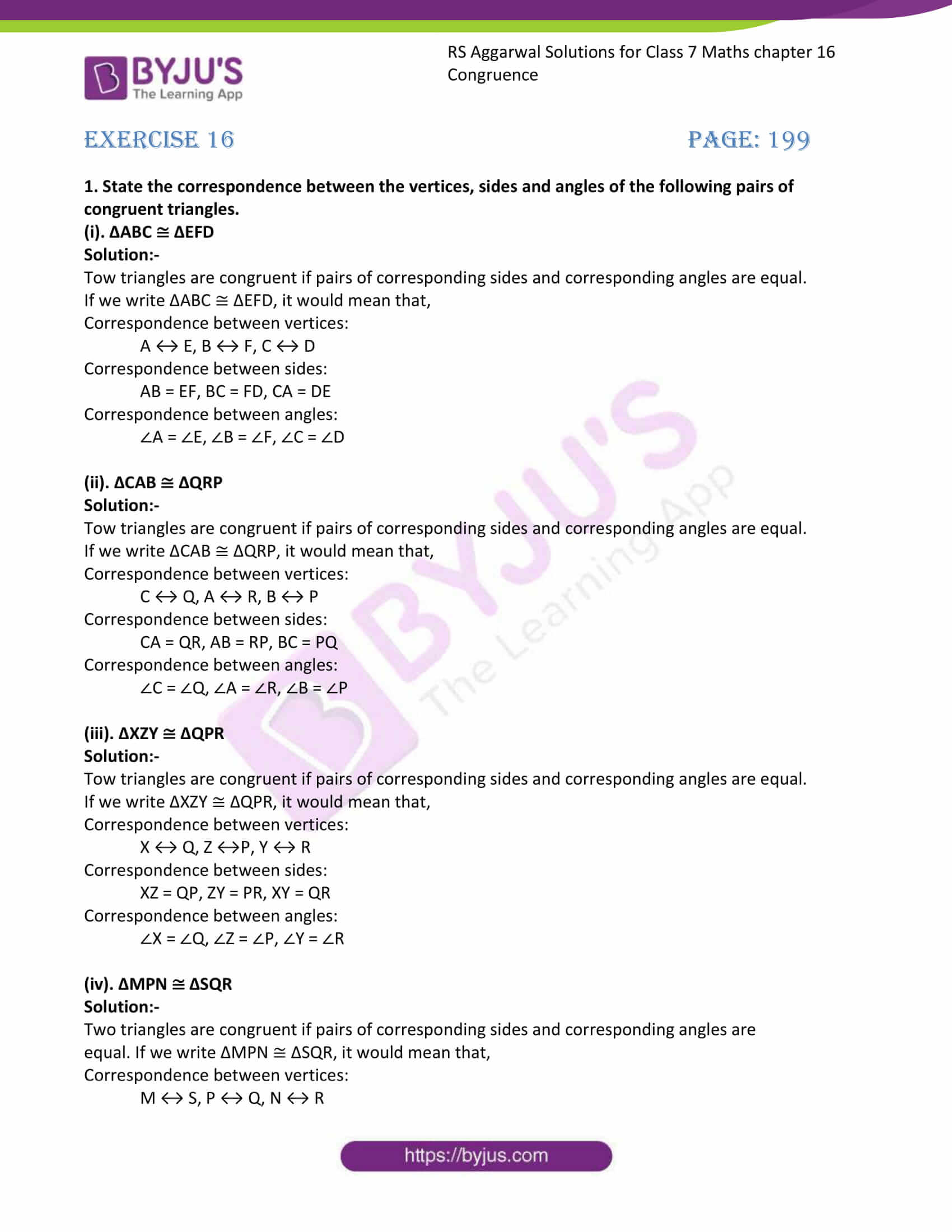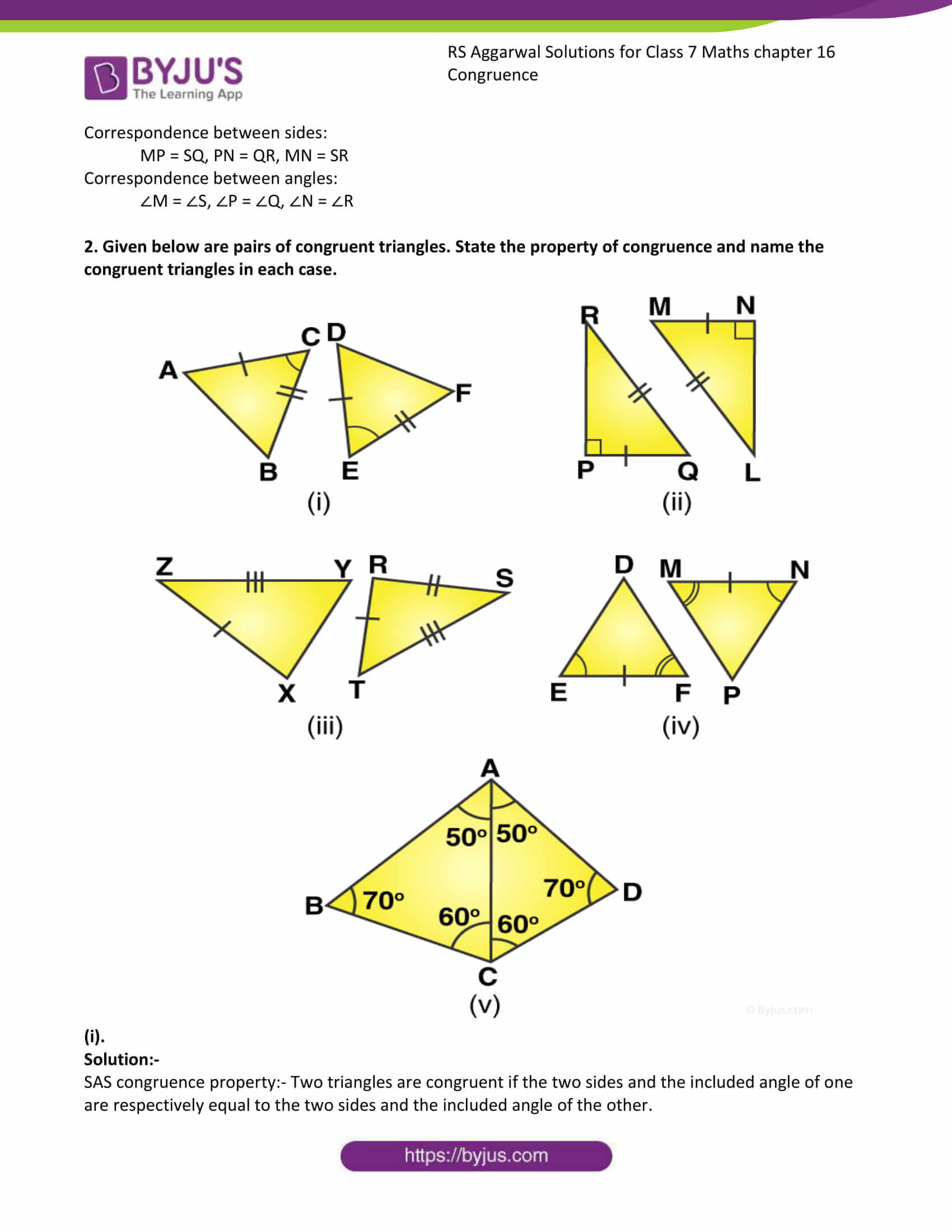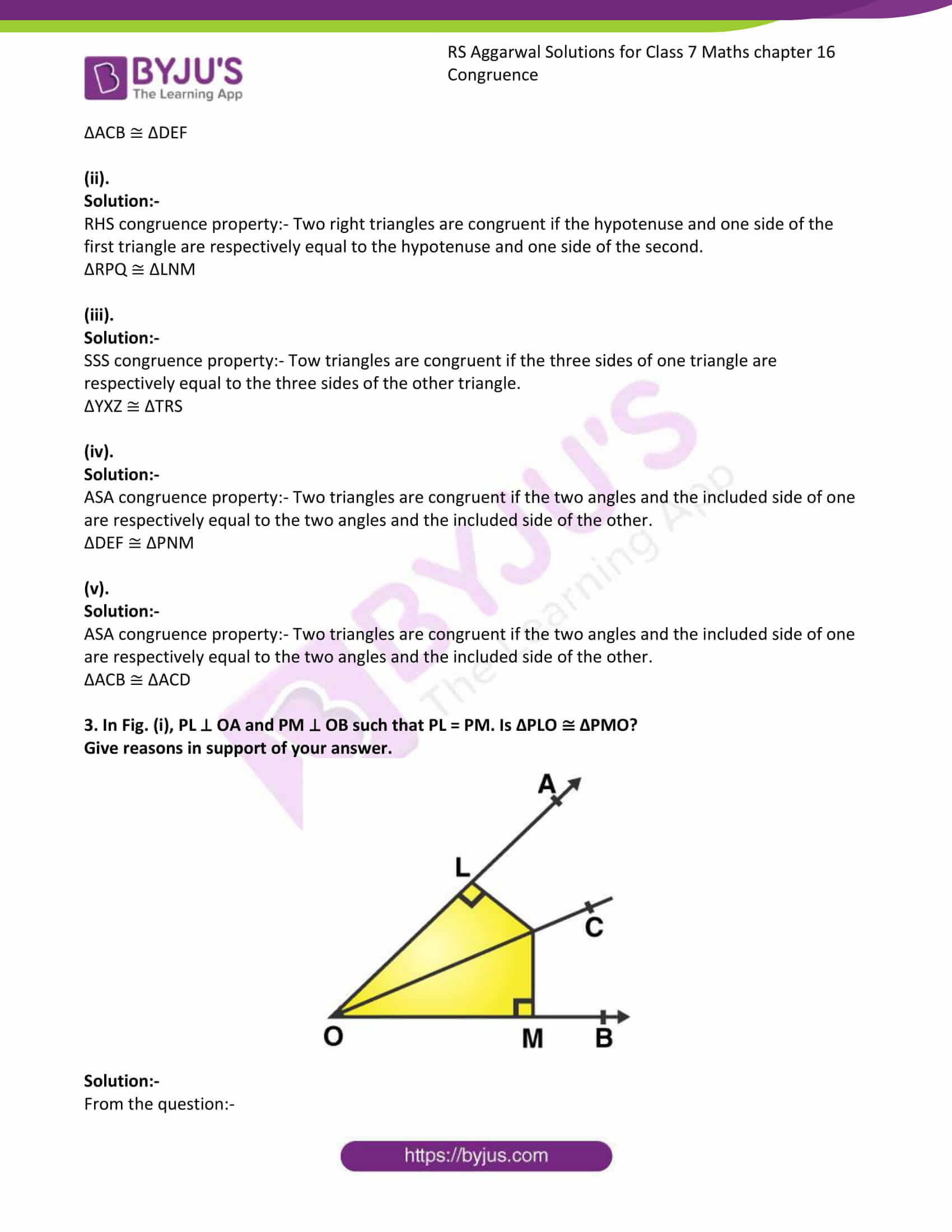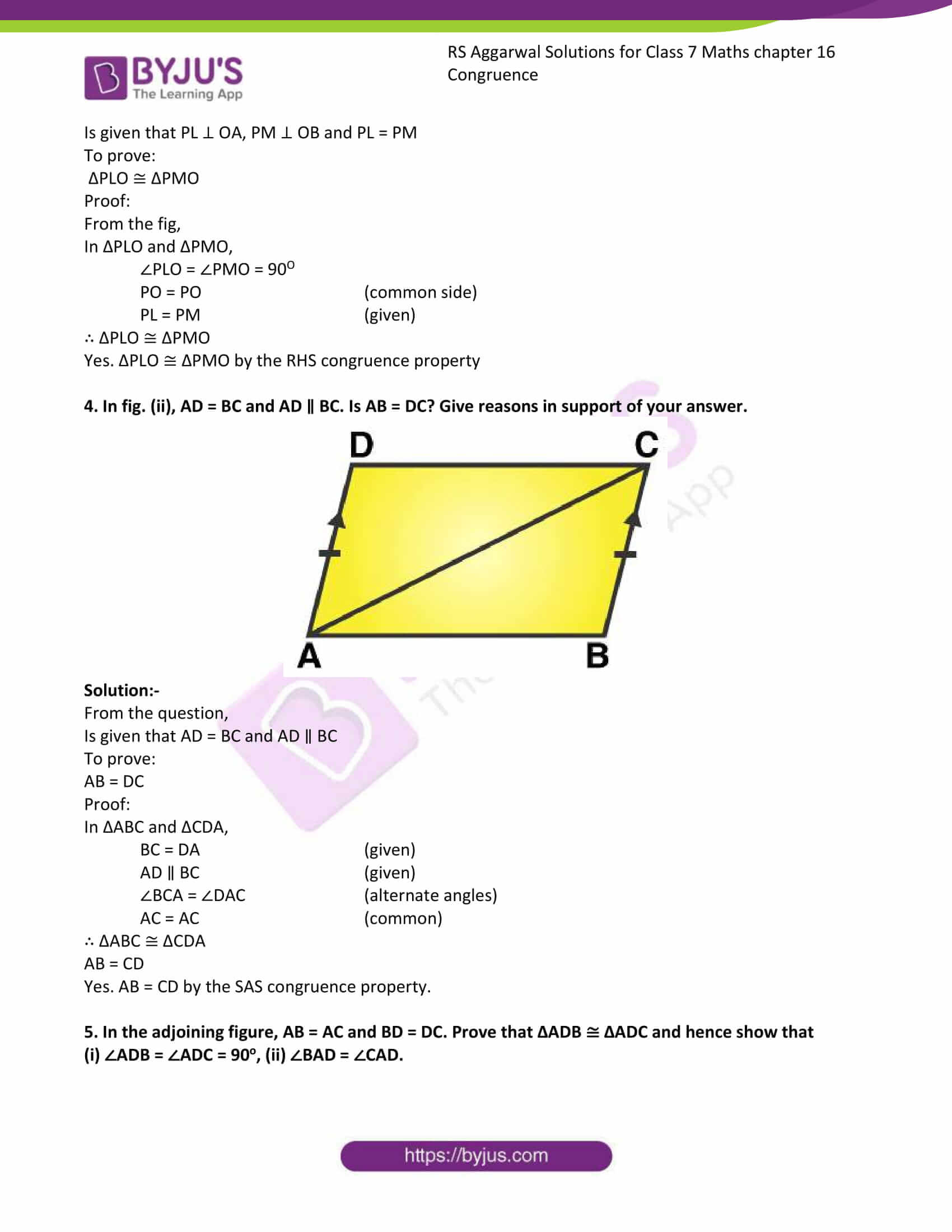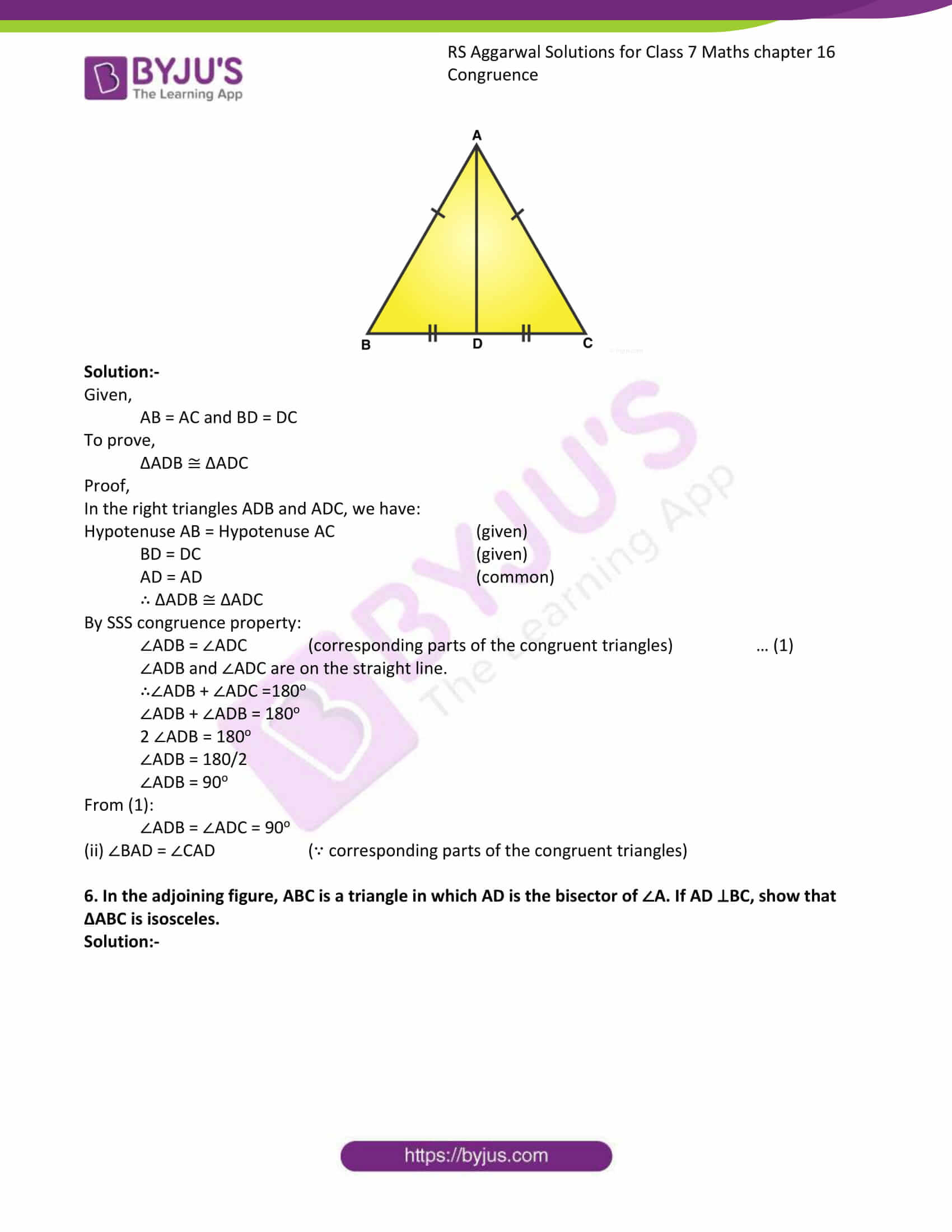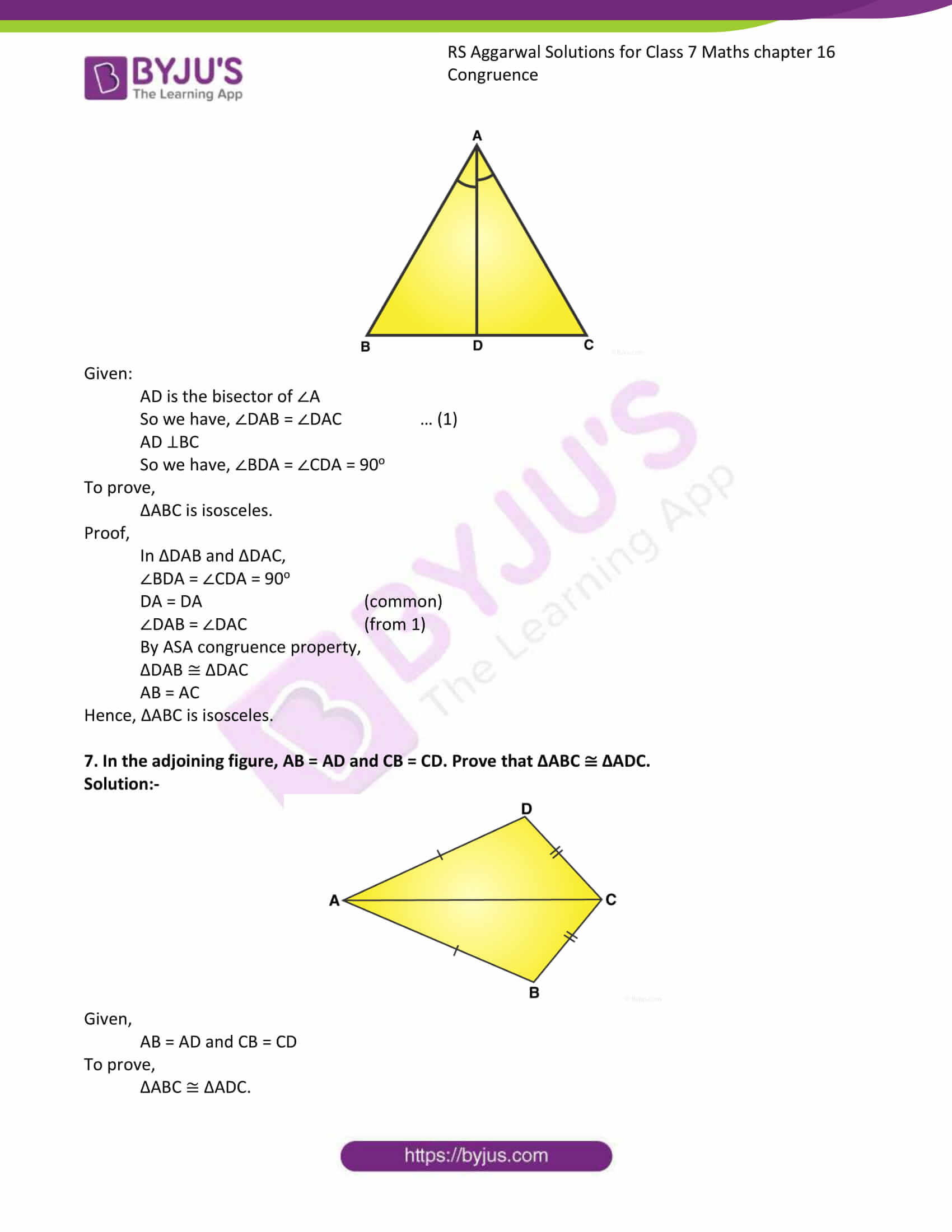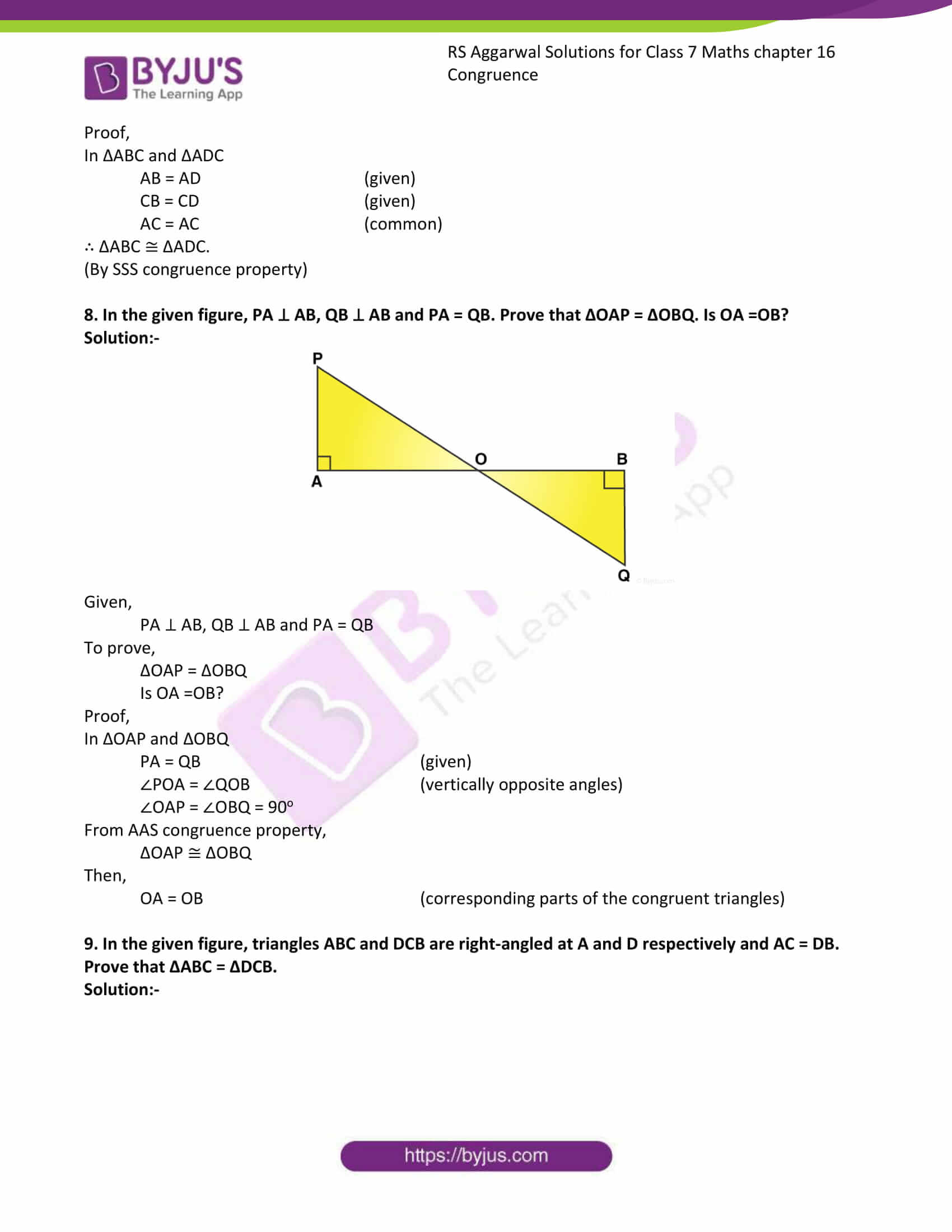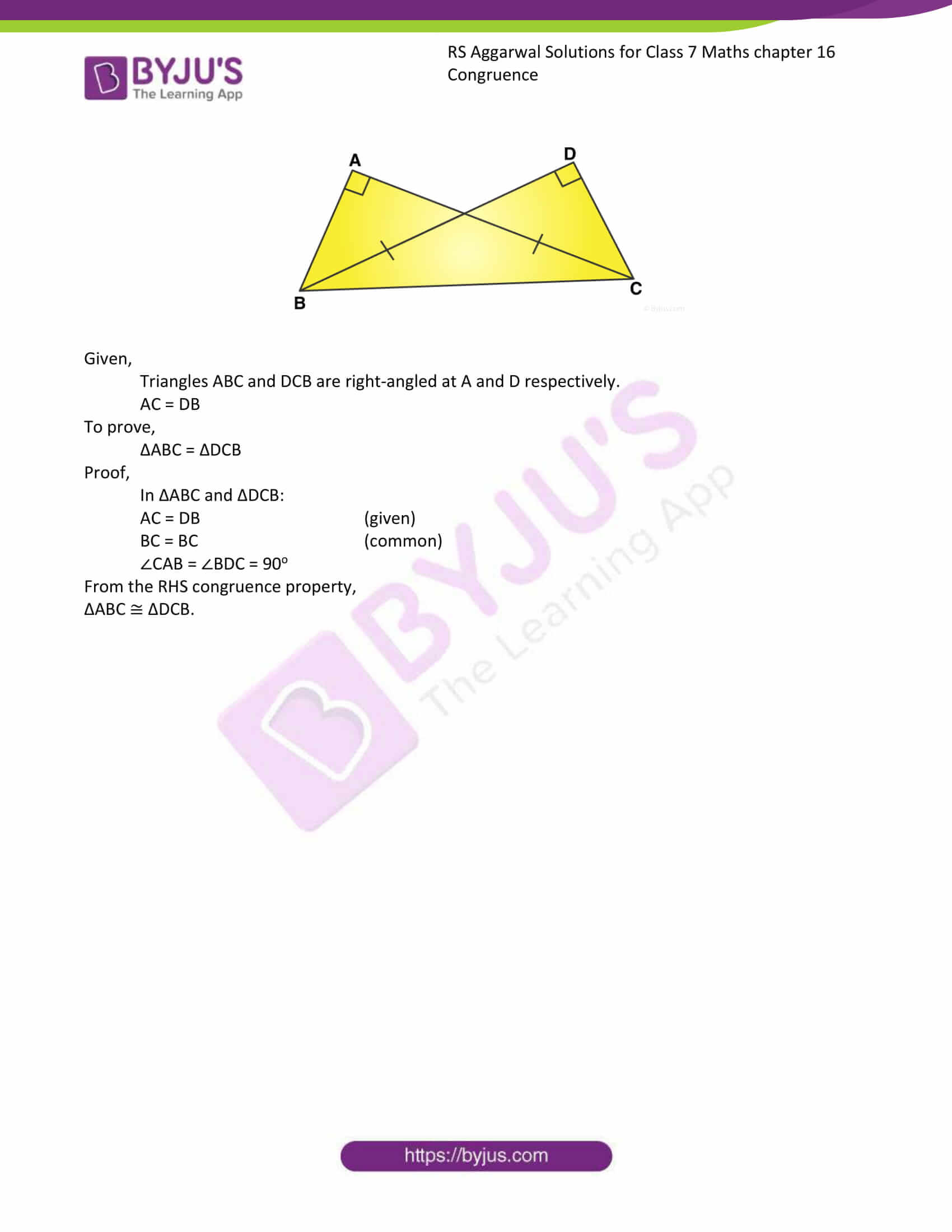### Access answer to chapter 16 – Congruence

Exercise 16 Page: 199

1. State the correspondence between the vertices, sides and angles of the following pairs of congruent triangles.

(i). ΔABC ≅ ΔEFD

Solution:-

Two triangles are congruent if pairs of corresponding sides and corresponding angles are equal.

If we write ΔABC ≅ ΔEFD, it would mean that,

Correspondence between vertices:

A ↔ E, B ↔ F, C ↔ D

Correspondence between sides:

AB = EF, BC = FD, CA = DE

Correspondence between angles:

∠A = ∠E, ∠B = ∠F, ∠C = ∠D

(ii). ΔCAB ≅ ΔQRP

Solution:-

Two triangles are congruent if pairs of corresponding sides and corresponding angles are equal.

If we write ΔCAB ≅ ΔQRP, it would mean that,

Correspondence between vertices:

C ↔ Q, A ↔ R, B ↔ P

Correspondence between sides:

CA = QR, AB = RP, BC = PQ

Correspondence between angles:

∠C = ∠Q, ∠A = ∠R, ∠B = ∠P

(iii). ΔXZY ≅ ΔQPR

Solution:-

Two triangles are congruent if pairs of corresponding sides and corresponding angles are equal.

If we write ΔXZY ≅ ΔQPR, it would mean that,

Correspondence between vertices:

X ↔ Q, Z ↔P, Y ↔ R

Correspondence between sides:

XZ = QP, ZY = PR, XY = QR

Correspondence between angles:

∠X = ∠Q, ∠Z = ∠P, ∠Y = ∠R

(iv). ΔMPN ≅ ΔSQR

Solution:-

Two triangles are congruent if pairs of corresponding sides and corresponding angles are equal.

If we write ΔMPN ≅ ΔSQR, it would mean that,

Correspondence between vertices:

M ↔ S, P ↔ Q, N ↔ R

Correspondence between sides:

MP = SQ, PN = QR, MN = SR

Correspondence between angles:

∠M = ∠S, ∠P = ∠Q, ∠N = ∠R

2. Given below are pairs of congruent triangles. State the property of congruence and name the congruent triangles in each case.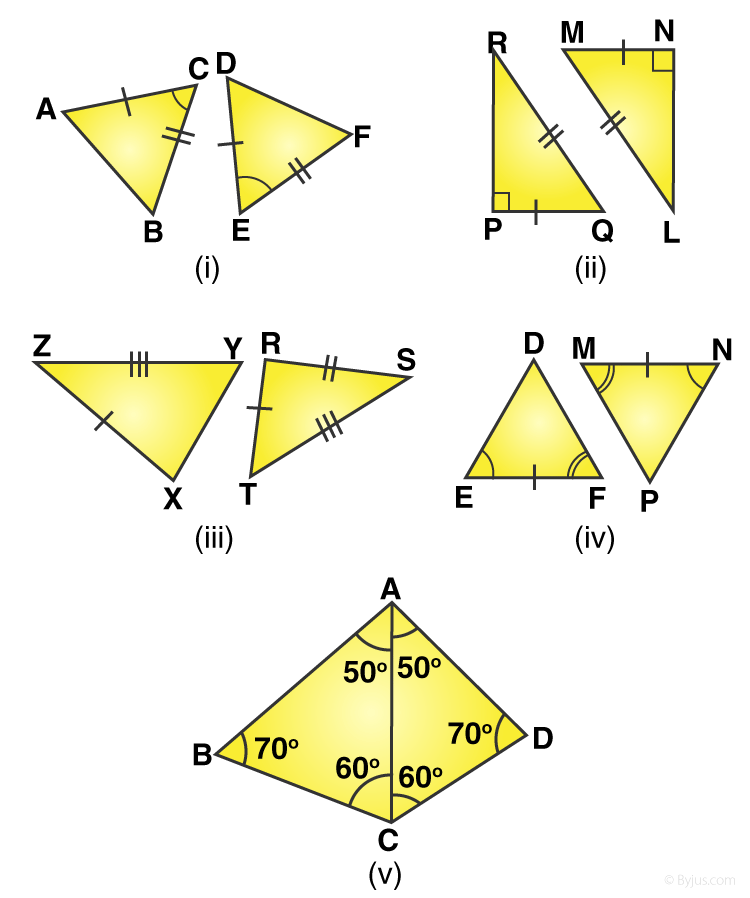(i).

Solution:-

SAS congruence property:- Two triangles are congruent if the two sides and the included angle of one are respectively equal to the two sides and the included angle of the other.

ΔACB ≅ ΔDEF

(ii).

Solution:-

RHS congruence property:- Two right triangles are congruent if the hypotenuse and one side of the first triangle are respectively equal to the hypotenuse and one side of the second.

ΔRPQ ≅ ΔLNM

(iii).

Solution:-

SSS congruence property:- Tow triangles are congruent if the three sides of one triangle are respectively equal to the three sides of the other triangle.

ΔYXZ ≅ ΔTRS

(iv).

Solution:-

ASA congruence property:- Two triangles are congruent if the two angles and the included side of one are respectively equal to the two angles and the included side of the other.

ΔDEF ≅ ΔPNM

(v).

Solution:-

ASA congruence property:- Two triangles are congruent if the two angles and the included side of one are respectively equal to the two angles and the included side of the other.

ΔACB ≅ ΔACD

3. In Fig. (i), PL ⊥ OA and PM ⊥ OB such that PL = PM. Is ΔPLO ≅ ΔPMO?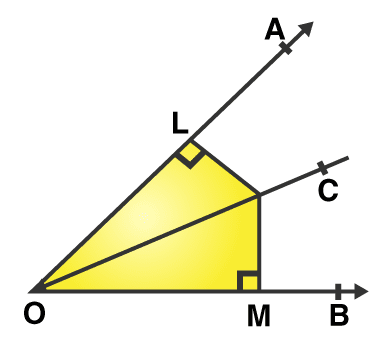Solution:-

From the question:-

Is given that PL ⊥ OA, PM ⊥ OB and PL = PM

To prove:

ΔPLO ≅ ΔPMO

Proof:

From the fig,

In ΔPLO and ΔPMO,

∠PLO = ∠PMO = 90O

PO = PO (common side)

PL = PM (given)

∴ ΔPLO ≅ ΔPMO

Yes. ΔPLO ≅ ΔPMO by the RHS congruence property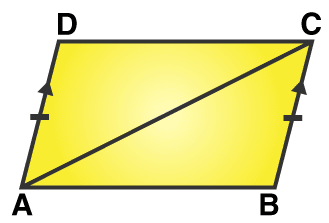Solution:-

From the question,

To prove:

AB = DC

Proof:

In ΔABC and ΔCDA,

BC = DA (given)

∠BCA = ∠DAC (alternate angles)

AC = AC (common)

∴ ΔABC ≅ ΔCDA

AB = CD

Yes. AB = CD by the SAS congruence property.

5. In the adjoining figure, AB = AC and BD = DC. Prove that ΔADB ≅ ΔADC and hence show that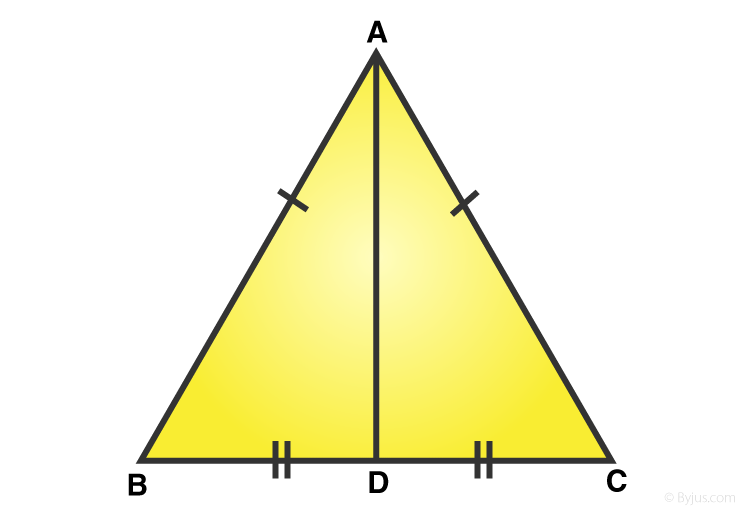Solution:-

Given,

AB = AC and BD = DC

To prove,

Proof,

Hypotenuse AB = Hypotenuse AC (given)

BD = DC (given)

By SSS congruence property:

From (1):

6. In the adjoining figure, ABC is a triangle in which AD is the bisector of ∠A. If AD ⊥BC, show that ΔABC is isosceles.

Solution:-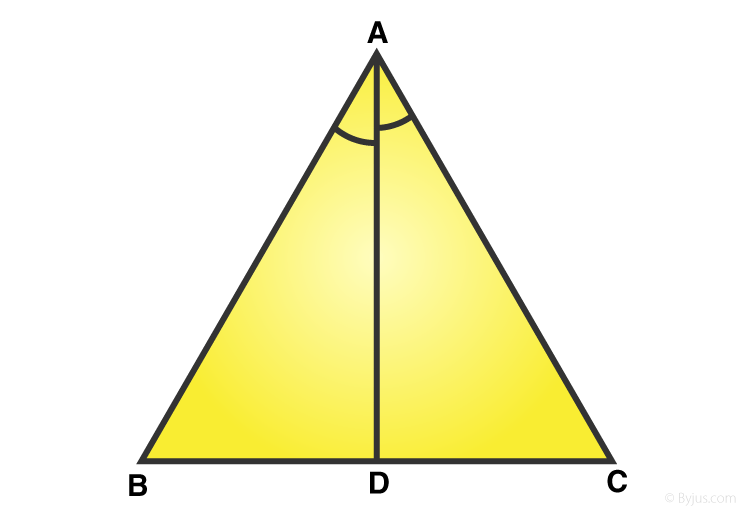Given:

AD is the bisector of ∠A

So we have, ∠DAB = ∠DAC … (1)

So we have, ∠BDA = ∠CDA = 90o

To prove,

ΔABC is isosceles.

Proof,

In ΔDAB and ΔDAC,

∠BDA = ∠CDA = 90o

DA = DA (common)

∠DAB = ∠DAC (from 1)

By ASA congruence property,

ΔDAB ≅ ΔDAC

AB = AC

Hence, ΔABC is isosceles.

7. In the adjoining figure, AB = AD and CB = CD. Prove that ΔABC ≅ ΔADC.

Solution:-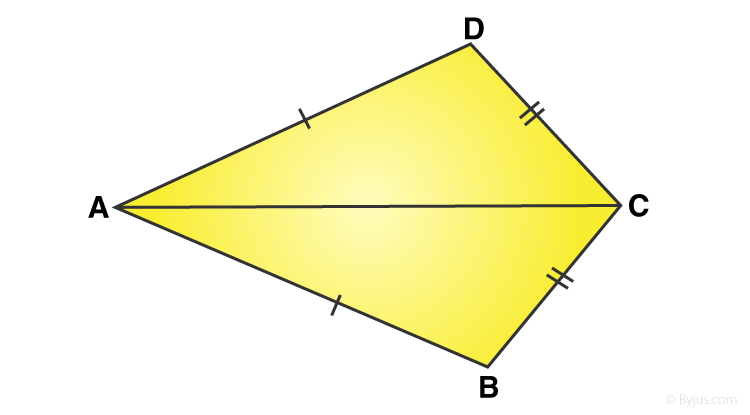Given,

AB = AD and CB = CD

To prove,

Proof,

CB = CD (given)

AC = AC (common)

(By SSS congruence property)

8. In the given figure, PA ⊥ AB, QB ⊥ AB and PA = QB. Prove that ΔOAP = ΔOBQ. Is OA =OB?

Solution:-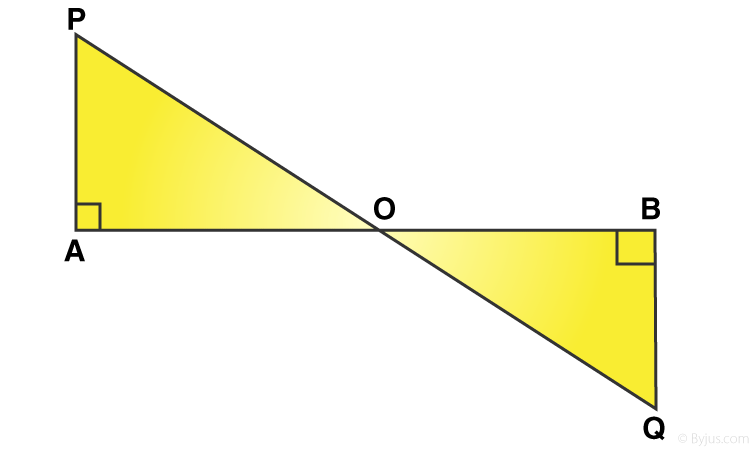Given,

PA ⊥ AB, QB ⊥ AB and PA = QB

To prove,

ΔOAP = ΔOBQ

Is OA =OB?

Proof,

In ΔOAP and ΔOBQ

PA = QB (given)

∠POA = ∠QOB (vertically opposite angles)

∠OAP = ∠OBQ = 90o

From AAS congruence property,

ΔOAP ≅ ΔOBQ

Then,

OA = OB (corresponding parts of the congruent triangles)

9. In the given figure, triangles ABC and DCB are right-angled at A and D respectively and AC = DB. Prove that ΔABC = ΔDCB.

Solution:-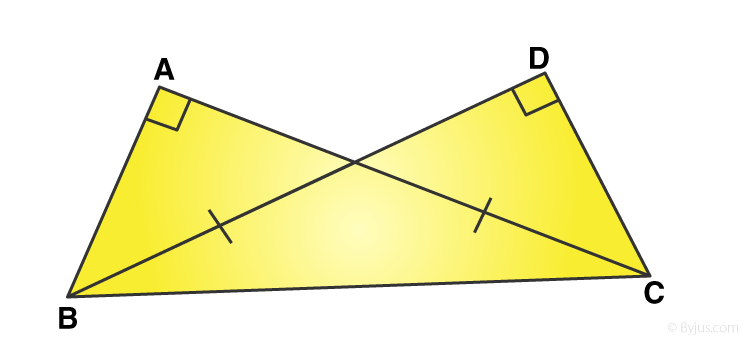Given,

Triangles ABC and DCB are right-angled at A and D respectively.

AC = DB

To prove,

ΔABC = ΔDCB

Proof,

In ΔABC and ΔDCB:

AC = DB (given)

BC = BC (common)

∠CAB = ∠BDC = 90o

From the RHS congruence property,

ΔABC ≅ ΔDCB.

## RS Aggarwal Solutions for Class 7 Maths Chapter 16 – Congruence

Chapter 16 – Congruence contains 1 exercise and the RS Aggarwal Solutions available on this page provide solutions to the questions present in the exercises. Now, let us have a look at some of the essential concepts discussed in this chapter.

• Congruent Figures
• Types of Congruent Figures
• Congruence of Triangles
• Congruence and Area

### Chapter Brief of RS Aggarwal Solutions for Class 7 Maths Chapter 16 – Congruence

RS Aggarwal Solutions for Class 7 Maths Chapter 16 – Congruence. Every figure has a shape, size and a position. If we are given two figures then by simply looking at them carefully we can decide whether they are of the same shape or not. The solutions are solved in such a way that students will understand clearly.